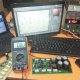Breaking News
Home / making transformer / how to make toroidal transformer?

# how to make toroidal transformer?

## how to make toroidal transformer:

Today we are going to learn how to make toroidal transformer? normally to making a transformer or constructing a transformer is very easy if you know that. here in this post, we can learn. toroidal transformer or classic transformer making rules are same.

At first, we can see the cour. we have to take core according to our watts. the transformer core size will be according to watts. such our amplifier will be 800 watts. so √800=28.28 so 28.28 X 28.28=800 Watts. then we have to take 20 square cm core. in this photo, we can see the core area is  7.8 cm. The 2nd and 3rd picture is for the measure core area. 4th picture is for using the cotton tape. this tape will protect the coil from the core. number 5th we can see spinning the primary coil. let’s go to calculate. wide is 1.5 cm and high is 5.2 cm. we know that wide X high = square. then 1.5 X 5.2=7.8cm. the core area is 7.8 cm. we know the transformer wire calculation rule is 42. this 42 is for all kinds of the transformer. 42 / 7.8=5.38. 5.38 turn coil need for one voltage. if our primary voltage is 220 then 220 X 5.38=1184.61 turn need. or our primary is 110 then 110 X 5.38=591.8 turn need.

Let’s go to watch the video for making or constructing a transformer.how to make toroidal transformer?

here in this video, we can see that how to make the transformer.

Now for the secondary, we need 35-0-35 voltage. For 1 voltage need 5.38 turn. so 35 X 5.38=188.46. we need center tap transformer so we need double secondary wire.

transistor circuit diagram of 2sa1943 and 2sc5200

Thanks a lot to be with us. If you want another post then please visit our website.

We have another post for you. like as repairing amplifier,